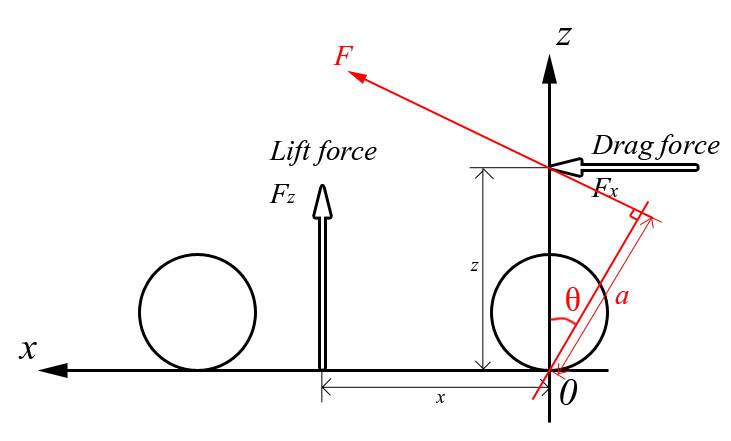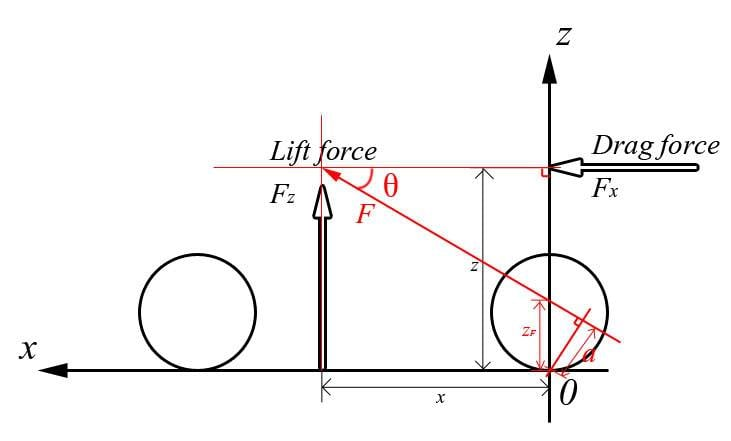# Aerodynamic forces acting upon a car body

Chob

## Homework Statement

I've performed a CFD analysis on a racecar model which has given my forces and torques (about the origin) in x,y,z. I'm struggling with the interpretation of those results. I'm interested in the point along the x axis about which the lift force acts.

Both forces are known, Torque about the origin due to these forces is known, the distances at which the forces act are the unknowns## Homework Equations

I would state this as Torque(about origin) = (Fxz) - (Fzx)

## The Attempt at a Solution

As far as I can tell there is 2 unknowns, too many to be solved with a single equation? This is a simplified version of my problem, the car is not symmetrical so there is also torque about the other 2 axis and I have tried to solve the three equations this sets up simultaneously but they are unsolvable, but I believe this is because the force in the y direction would not necessarily act at the same point along the x axis as the force in the z direction. But then putting extra terms in for the different distances means 3 equations and 6 unknowns which leaves the same problem so I'm back to this simplified version.

Chob
I've been thinking about it a bit more and come up with this.The resultant F can be worked out by Pythagoras and angle theta from Theta = arctan (Fz/Fx), and distance 'a' can be had from Torque = Force x distance, I can then use Cos(Theta) = (a/z) to find z and from there I've only one unknown ?

Chob
Okay I've drawn that red arrow in the wrong place I'm sure. I think it should be like thisOnly problem is I can't see how to get z or x out, there's still two unknowns. I drew the force arrow for lift too long (Fz) it's actually lesser than Fx.

Gold Member
Welcome to PF!

You would normally model the two forces as acting through the center of mass (CM) of the vehicle, so I assume that (x,z) is the CM of the vehicle in your problem. Next you should probably consider if you got the direction of the forces right, especially the drag force which should point in the same direction as the relative wind vector. Once you have your two vectors in order, you can apply them to the CM to get the total force (vector sum of the two).

The torque can now be calculated in a number of ways. If you have the vectors as numbers it is probably easiest to simply apply the vector product between the total force through CM with the displacement vector between CM and the torque axis (see for instance  for a diagram and equations).

 http://en.wikipedia.org/wiki/Torque, Definition and relation to angular momentum

Chob
Hello, thanks for the reply. I don't have the centre of mass of the vehicle, it's just a CAD model at the moment with no material properties applied. x and z are the unknowns, x is the one I particularly want to find as it will allow me to find the reaction forces on the front and rear axle. I have the Torque and force values from my CFD simulation.

Chob
Actually that's given me a thought, I think perhaps I should be looking for the point on the car about which there aren't any moments? in which case the red arrow should pass through the origin? I still don't think that allows me to find the size of x and z, just the ratio between them :/

Gold Member
Perhaps you could detail your model some more and describe what kind of questions you want your analysis to answer?

Reading your description so far leaves me with some questions that may be relevant in understanding your problem: why is the weight of the car not included in the model? If the CFD model consist of several elements for the car or chassis, how did you sum the forces and torques? Is the x and z the center of pressure from the CFD tool? Why do you want to know the torque around what appears to be the bottom of one of the wheel pairs? Is the car moving relative to the ground and if so, is it accelerating? Are the wheels torqued by engine or brakes? How much sense will a (numerial) analysis make if you do not know the material properties? If this is homework, what is the assignment?

Chob
Sorry it's not clear, I appreciate you taking the time to try and help, I'll try and answer your questions in order.

The weight of the car is not in my control, I am only interested in aerodynamic forces.

Total force in x,y,z and total torque in x,y,z are what the CFD software calculates, it does not have an output for the centre of pressure, I'm new to aerodynamic terminology but looking this up this seems to be the axis the car will pitch about and where the downforce will act through, this is what I want to solve.

I am not interested in the Torque at contact patch of the front wheels, along the centre-line of the car is where the origin (0,0,0) is set on the model, this is the point the CFD software uses when reporting the torques.

The simulation takes the car moving at a constant velocity, the model has no moving parts.

It's not a set assignment but rather a project to optimise the aerodynamic performance of the car, to do this I intend to attempt at reducing the drag Force and creating a negative lift, finding a good trade-off between the two. I can already do this from the total force in x and z but it seems to me I should also be investigating the point through which the down-force is applied and not just it's magnitude for balance reasons.

Gold Member
Good descriptions there, Chob.

... it seems to me I should also be investigating the point through which the down-force is applied and not just it's magnitude for balance reasons.

In general in statics, if you have force FA and torque NA about point A, you can change to another reference point B by observing that

FB = FA, and
NB = NA + (A-B) x FA.

In your case, you can for instance use this to find the center of pressure point CP where NCP = 0 or, using A = 0, where CP x F = N with F and N from your CFD tool. The position of CP relative to the geometry of the car should give you an indication if the static aerodynamic forces are "balanced" for a particular car shape. For dynamic stability you want your CP a bit aft and above CM, but since you don't have the CM exactly you may just estimate the area it will be in based on symmetry, engine location and such.